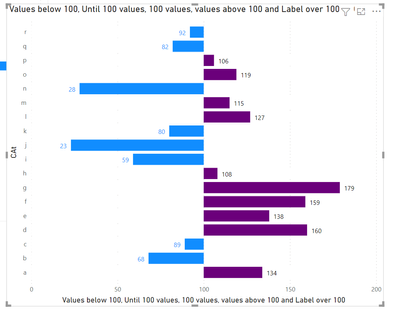cancel
Showing results for
Did you mean:Regular Visitor

## Horizontal bar charts with custom center line

Hi everyone,

I'm trying to replicate in Power BI a visual I had no problems creating in excel.

It's a horizontal bar chart where the center line on the x axis is 100. If a value is over 100 the bar goes right, otherwise it goes left (See below)From what I've found reading other threads I didn't find a solution: it seems in Power BI there's no equivalent option to that "vertical axis crosses" you see in excel. I've tried a workaround using the tornado visual but it doesn't suit my needs, since the bars diverge differently.

Does anybody have any idea on how to solve this?

Thanks,

Gianluca

1 ACCEPTED SOLUTIONSuper User

You can do this by making some measures and tweaking the chart in order to have the correct values.

Create the following measures:

``````Values below 100 =
IF(SUM('Table (2)'[VAlue]) <= 100 , SUM('Table (2)'[VAlue]))

Until 100 values = IF(SUM('Table (2)'[VAlue])<= 100, 100-SUM('Table (2)'[VAlue]))

100 values = IF(SUM('Table (2)'[VAlue])> 100, 100)

values above 100 = if(SUM('Table (2)'[VAlue]) > 100 , SUM('Table (2)'[VAlue]) -100 )

Label over 100 = if(SUM('Table (2)'[VAlue]) > 100, SUM('Table (2)'[VAlue]))``````

Now create a stacked bar chart and place the measures in the following order:

Values below 100

Until 100 values

100 Values

values above 100

label over 100

Now on the setup of your chart do the following:

Colors of the measure below should be white (or equal to background)

values below 100

100 values

label over 100

data labels for all of the measure below should be turn off:

until 100 values

values above 100

100 values

Labels for the measure below should be place on specified location:

values below 100 - inside end

Label over 100 - inside start

Set the x-axis maximum value to 100

You should also do a custom tooltip instead of the normal one.

Result below and in attach file.Regards

Miguel Félix

Proud to be a Super User!

Check out my blog: Power BI em PortuguêsSuper User

You can do this by making some measures and tweaking the chart in order to have the correct values.

Create the following measures:

``````Values below 100 =
IF(SUM('Table (2)'[VAlue]) <= 100 , SUM('Table (2)'[VAlue]))

Until 100 values = IF(SUM('Table (2)'[VAlue])<= 100, 100-SUM('Table (2)'[VAlue]))

100 values = IF(SUM('Table (2)'[VAlue])> 100, 100)

values above 100 = if(SUM('Table (2)'[VAlue]) > 100 , SUM('Table (2)'[VAlue]) -100 )

Label over 100 = if(SUM('Table (2)'[VAlue]) > 100, SUM('Table (2)'[VAlue]))``````

Now create a stacked bar chart and place the measures in the following order:

Values below 100

Until 100 values

100 Values

values above 100

label over 100

Now on the setup of your chart do the following:

Colors of the measure below should be white (or equal to background)

values below 100

100 values

label over 100

data labels for all of the measure below should be turn off:

until 100 values

values above 100

100 values

Labels for the measure below should be place on specified location:

values below 100 - inside end

Label over 100 - inside start

Set the x-axis maximum value to 100

You should also do a custom tooltip instead of the normal one.

Result below and in attach file.Regards

Miguel Félix

Proud to be a Super User!

Check out my blog: Power BI em Português